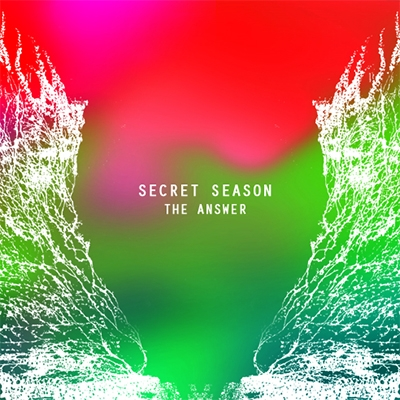# The equation

Creation, variation,
all part of the equation

part of the equation

Every lapse of concentration

Every piece of information
Part of the equation

Signals fly station to station
Part of the equation

Rise and fall of a great nation

Every star and constellation
Part of the equation